# Treasure Hunt SequencesPage 1

#### WATCH ALL SLIDES

Slide 1## Mathematical Treasure-hunt: Sequences

Cut out each of the question slides and place them around the room, stick them on the walls if you wish.

Print out and distribute the answer sheet, one per pupil, or team, and set them off to find the answers.

Slide 2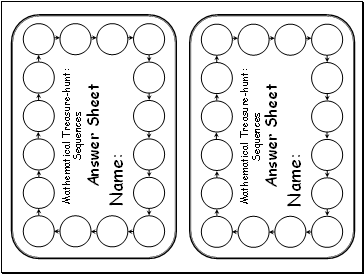Mathematical Treasure-hunt: Sequences

Name:

Mathematical Treasure-hunt: Sequences

Name:

Slide 3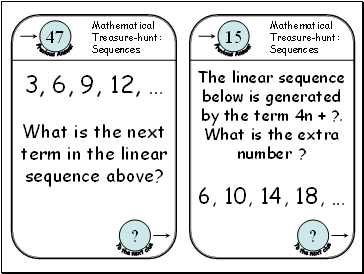3, 6, 9, 12, …

What is the next term in the linear sequence above?

6, 10, 14, 18, …

The linear sequence below is generated by the term 4n + ?. What is the extra number ?

Slide 429, 22, 15, 8, …

What is the 5th term, U5, in the linear sequence below?

1, 1, 2, 3, 5, 8, 13, 21, …

Consider the Fibonacci sequence below:

What is the Square Root of the 12th term, U12 ?

Slide 5The rule for generating a sequence is:

“Add four on to the previous term.”

If the first term is 1, what is the third term, U3?

1, 3, 6, 10, 15, 21, …

How many sides does the shape associated with this sequence have?

Slide 6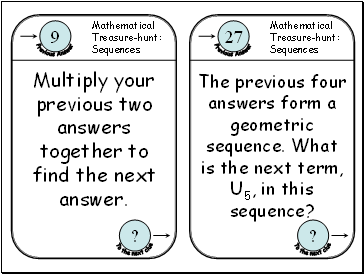The previous four answers form a geometric sequence. What is the next term, U5, in this sequence?

Slide 7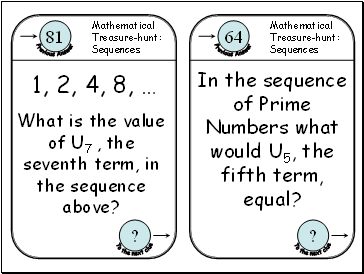1, 2, 4, 8, …

What is the value of U7 , the seventh term, in the sequence above?

In the sequence of Prime Numbers what would U5, the fifth term, equal?

Slide 83, 7, 11, 15, …

What is the common difference in this linear sequence?

The product of your previous two answers should equal 44. If this is the second term, U2, in a sequence with a common difference of -10 what would U1, the first term, be?

Slide 9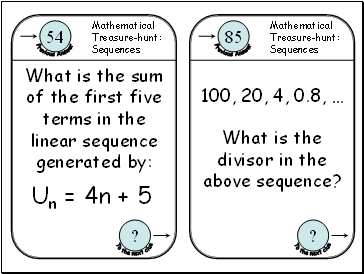Un = 4n + 5

What is the sum of the first five terms in the linear sequence generated by:

100, 20, 4, 0.8, …

What is the divisor in the above sequence?

Slide 10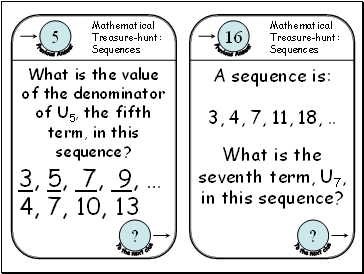3, 5, 7, 9, …

4, 7, 10, 13

What is the value of the denominator of U5, the fifth term, in this sequence?

3, 4, 7, 11, 18,

What is the seventh term, U7, in this sequence?

A sequence is:

Go to page:
1  2